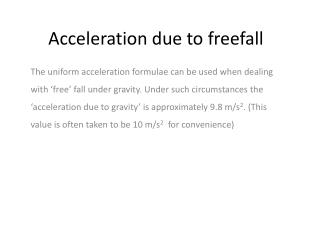Download PresentationAcceleration due to freefall

# Acceleration due to freefall - PowerPoint PPT PresentationDownload Presentation## Acceleration due to freefall

- - - - - - - - - - - - - - - - - - - - - - - - - - - E N D - - - - - - - - - - - - - - - - - - - - - - - - - - -
##### Presentation Transcript

1. Acceleration due to freefall The uniform acceleration formulae can be used when dealing with ‘free’ fall under gravity. Under such circumstances the ‘acceleration due to gravity’ is approximately 9.8 m/s2. (This value is often taken to be 10 m/s2 for convenience)

2. Example A brick is thrown vertically downwards from the top of a building and has an initial velocity of 1.5m/s. If the height of the building is 20m, taking g = 10 m/s2 find a) The velocity with which the brick hits the ground, b) The time taken for the brick to fall.

3. Example A ball is thrown vertically upwards with a speed of 14.7 m/s from a platform 19.6m above level ground. Calculate, a) The time taken for the ball to reach the ground b) The speed of the ball when it reaches the ground.

4. Example A particle is projected vertically upwards with a speed of 34.3 m/s. Find how long after projection the particle is a) At its maximum height b) At a height of 49m above the point of projection for (i) the first time (ii) the second time.

5. Example A ball is thrown vertically upwards with a velocity of 34.3m/s. Find how long after projection the particle is at a height of 49m above the point of projection for a) The first time b) the second time.

6. 1.A level past paper question • A ball is thrown vertically upwards from the ground with initial speed 11.2 m/s. • Find the greatest height reached by the ball. • Calculate the length of time the ball is more than 2.8m above the ground. • State one assumption you have made in your solution of the problem.